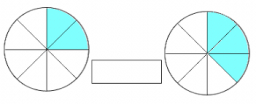What is similar fraction

Which of the following is the similar fraction of 9/10,3/5,1/4

s =  7/12

Step-by-step explanation:Did you find an error or inaccuracy? Feel free to write us. Thank you!Tips to related online calculators
Looking for help with calculating arithmetic mean?
Looking for a statistical calculator?
Need help to calculate sum, simplify or multiply fractions? Try our fraction calculator.

You need to know the following knowledge to solve this word math problem:

We encourage you to watch this tutorial video on this math problem: